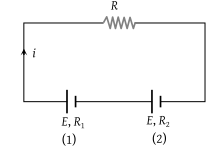NEET Questions Solved

Two sources of equal emf are connected to an external resistance R. The internal resistances of the two sources are R1 and ${R}_{2}\text{\hspace{0.17em}}\left({R}_{2}>{R}_{1}\right)$. If the potential difference across the source having internal resistance R2 is zero, then

(1) $R={R}_{1}{R}_{2}/\left({R}_{1}+{R}_{2}\right)$

(2) $R={R}_{1}{R}_{2}/\left({R}_{2}-{R}_{1}\right)$

(3) $R={R}_{2}×\left({R}_{1}+{R}_{2}\right)/\left({R}_{2}-{R}_{1}\right)$

(4) $R={R}_{2}-{R}_{1}$

(4) $i=\frac{2E}{R+{R}_{1}+{R}_{2}}$

From cell (2) $E=V+i{R}_{2}=0+i{R}_{2}$$E=\frac{2E}{R+{R}_{1}+{R}_{2}}×{R}_{2}$$R={R}_{2}-{R}_{1}$

Difficulty Level:

• 11%
• 42%
• 18%
• 32%